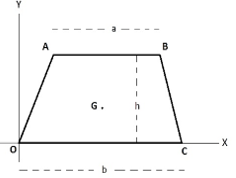# Centroid of a Trapezoid Formula

## Centroid of a Trapezoid Formulas - Find the Centroid of a Trapezium

Consider the following trapezium in which AB || CD. The trapezium OABC is placed such that the origin coincides with one of its vertices. G is its centroid. The lengths of its parallel sides are AB = a and OC = b and its height is h.The coordinates of the centroid of the trapezium are given by the following formula.
$G\left( {\frac{h}{2},\,\frac{{b + 2a}}{{3\left( {a + b} \right)}}h} \right)$
Let’s look at an example to see how to use this formula.

Question: Find the centroid of a trapezium of height 5 cm whose parallel sides are 6 cm and 8 cm.
Solution:
$a = 6\,cm,\,\,b = 8\,cm,\,\,h = 5\,cm$
${G_y} = \frac{{b + 2a}}{{3\left( {a + b} \right)}}h = \frac{{8 + 12}}{{3\left( {8 + 6} \right)}} \times 5 = \frac{{20 \times 5}}{{42}} = 2.38\,cm$
Hence, the centroid is 2.38 cm from the side whose length is 8 cm.

Why don’t you try to solving a problem for practice?

Question: Find the centroid of a trapezium of height 4.5 cm whose parallel sides are 4 cm and 8 cm.
Options:
(a) 2 cm from the side whose length is 4 cm
(b) 2 cm from the side whose length is 8 cm
(c) 3 cm from the side whose length is 4 cm
(d) 3 cm from the side whose length is 8 cm
$a = 4\,cm,\,\,b = 8\,cm,\,\,h = 4.5\,cm$
${G_y} = \frac{{b + 2a}}{{3\left( {a + b} \right)}}h = \frac{{8 + 8}}{{3\left( {8 + 4} \right)}} \times 4.5 = \frac{{16 \times 4.5}}{{3 \times 12}} = \frac{{72}}{{36}} = 2\,cm$